# Data Presentation using Descriptive Graphs.pptx

19 de Mar de 2022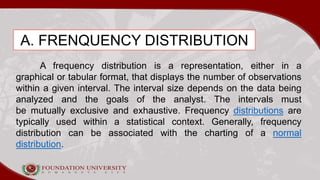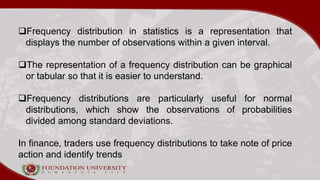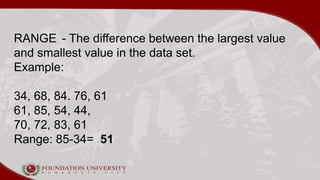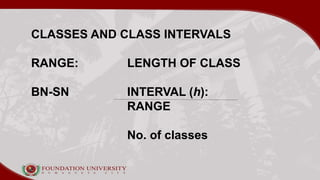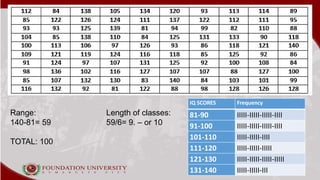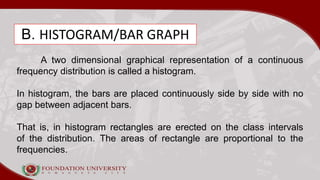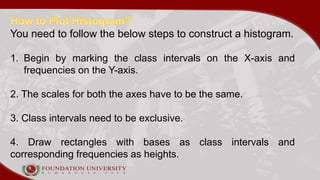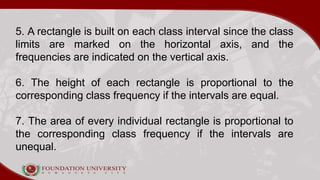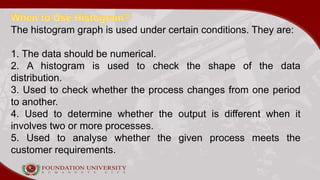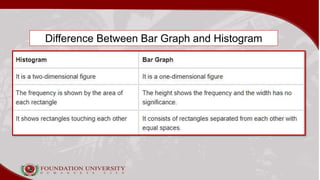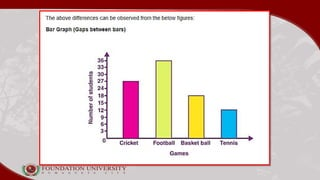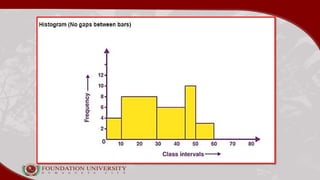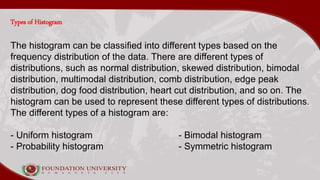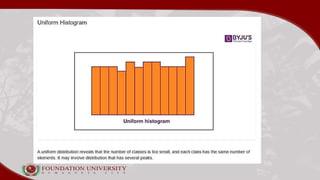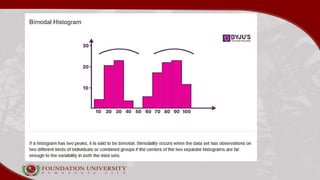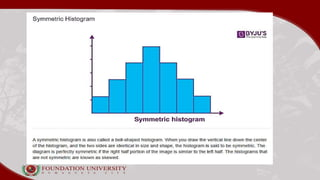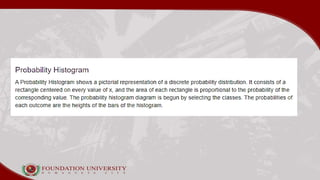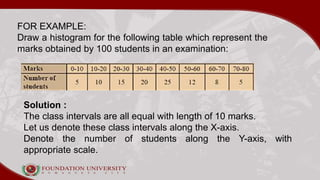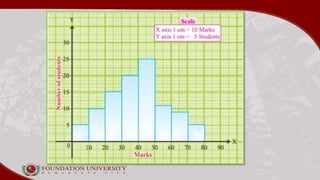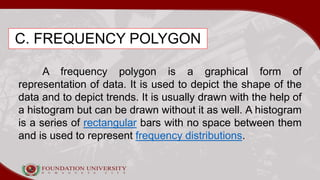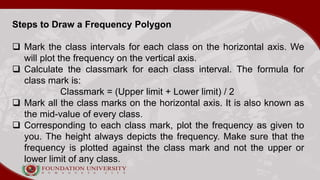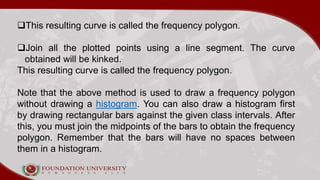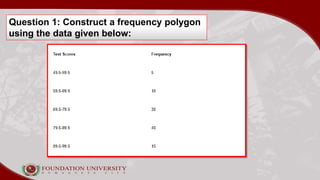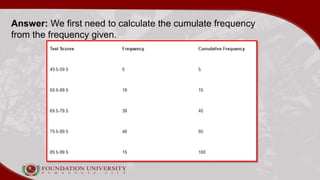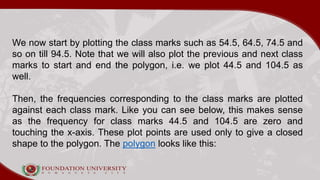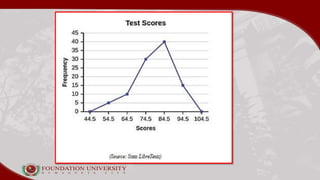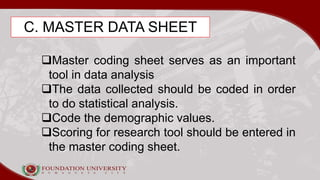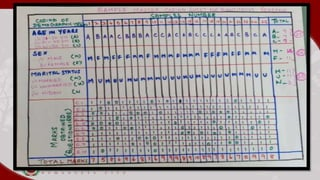1 de 32

### Data Presentation using Descriptive Graphs.pptx

• 3. A. FRENQUENCY DISTRIBUTION A frequency distribution is a representation, either in a graphical or tabular format, that displays the number of observations within a given interval. The interval size depends on the data being analyzed and the goals of the analyst. The intervals must be mutually exclusive and exhaustive. Frequency distributions are typically used within a statistical context. Generally, frequency distribution can be associated with the charting of a normal distribution.
• 4. Frequency distribution in statistics is a representation that displays the number of observations within a given interval. The representation of a frequency distribution can be graphical or tabular so that it is easier to understand. Frequency distributions are particularly useful for normal distributions, which show the observations of probabilities divided among standard deviations. In finance, traders use frequency distributions to take note of price action and identify trends
• 5. RANGE - The difference between the largest value and smallest value in the data set. Example: 34, 68, 84. 76, 61 61, 85, 54, 44, 70, 72, 83, 61 Range: 85-34= 51
• 6. CLASSES AND CLASS INTERVALS RANGE: LENGTH OF CLASS BN-SN INTERVAL (h): RANGE No. of classes
• 7. IQ SCORES Frequency 81-90 IIIII-IIIII-IIIII-IIII 91-100 IIIII-IIIII-IIIII-IIII 101-110 IIIII-IIIII-IIII 111-120 IIIII-IIIII-IIIII 121-130 IIIII-IIIII-IIIII-IIIII 131-140 IIIII-IIIII-III Range: Length of classes: 140-81= 59 59/6= 9. – or 10 TOTAL: 100
• 8. B. HISTOGRAM/BAR GRAPH A two dimensional graphical representation of a continuous frequency distribution is called a histogram. In histogram, the bars are placed continuously side by side with no gap between adjacent bars. That is, in histogram rectangles are erected on the class intervals of the distribution. The areas of rectangle are proportional to the frequencies.
• 9. You need to follow the below steps to construct a histogram. 1. Begin by marking the class intervals on the X-axis and frequencies on the Y-axis. 2. The scales for both the axes have to be the same. 3. Class intervals need to be exclusive. 4. Draw rectangles with bases as class intervals and corresponding frequencies as heights.
• 10. 5. A rectangle is built on each class interval since the class limits are marked on the horizontal axis, and the frequencies are indicated on the vertical axis. 6. The height of each rectangle is proportional to the corresponding class frequency if the intervals are equal. 7. The area of every individual rectangle is proportional to the corresponding class frequency if the intervals are unequal.
• 11. The histogram graph is used under certain conditions. They are: 1. The data should be numerical. 2. A histogram is used to check the shape of the data distribution. 3. Used to check whether the process changes from one period to another. 4. Used to determine whether the output is different when it involves two or more processes. 5. Used to analyse whether the given process meets the customer requirements.
• 12. Difference Between Bar Graph and Histogram
• 15. Types of Histogram The histogram can be classified into different types based on the frequency distribution of the data. There are different types of distributions, such as normal distribution, skewed distribution, bimodal distribution, multimodal distribution, comb distribution, edge peak distribution, dog food distribution, heart cut distribution, and so on. The histogram can be used to represent these different types of distributions. The different types of a histogram are: - Uniform histogram - Bimodal histogram - Probability histogram - Symmetric histogram
• 20. FOR EXAMPLE: Draw a histogram for the following table which represent the marks obtained by 100 students in an examination: Solution : The class intervals are all equal with length of 10 marks. Let us denote these class intervals along the X-axis. Denote the number of students along the Y-axis, with appropriate scale.
• 22. C. FREQUENCY POLYGON A frequency polygon is a graphical form of representation of data. It is used to depict the shape of the data and to depict trends. It is usually drawn with the help of a histogram but can be drawn without it as well. A histogram is a series of rectangular bars with no space between them and is used to represent frequency distributions.
• 23. Steps to Draw a Frequency Polygon  Mark the class intervals for each class on the horizontal axis. We will plot the frequency on the vertical axis.  Calculate the classmark for each class interval. The formula for class mark is: Classmark = (Upper limit + Lower limit) / 2  Mark all the class marks on the horizontal axis. It is also known as the mid-value of every class.  Corresponding to each class mark, plot the frequency as given to you. The height always depicts the frequency. Make sure that the frequency is plotted against the class mark and not the upper or lower limit of any class.
• 24. This resulting curve is called the frequency polygon. Join all the plotted points using a line segment. The curve obtained will be kinked. This resulting curve is called the frequency polygon. Note that the above method is used to draw a frequency polygon without drawing a histogram. You can also draw a histogram first by drawing rectangular bars against the given class intervals. After this, you must join the midpoints of the bars to obtain the frequency polygon. Remember that the bars will have no spaces between them in a histogram.
• 25. Question 1: Construct a frequency polygon using the data given below:
• 26. Answer: We first need to calculate the cumulate frequency from the frequency given.
• 27. We now start by plotting the class marks such as 54.5, 64.5, 74.5 and so on till 94.5. Note that we will also plot the previous and next class marks to start and end the polygon, i.e. we plot 44.5 and 104.5 as well. Then, the frequencies corresponding to the class marks are plotted against each class mark. Like you can see below, this makes sense as the frequency for class marks 44.5 and 104.5 are zero and touching the x-axis. These plot points are used only to give a closed shape to the polygon. The polygon looks like this:
• 29. C. MASTER DATA SHEET Master coding sheet serves as an important tool in data analysis The data collected should be coded in order to do statistical analysis. Code the demographic values. Scoring for research tool should be entered in the master coding sheet.